TitleCollege Algebra
Tutorial 44: Logarithmic
PropertiesLearning Objectives

 After completing this tutorial, you should be able to: Be familiar with and use properties of logarithms in various situations.Introduction

 In this tutorial I am going to help you expand your knowledge of logarithms.  Probably the biggest thing you need to remember to help you out with this section is that LOGS ARE ANOTHER WAY TO WRITE EXPONENTS.  If you keep that little tidbit of information at the forefront of your brain, that will help you out TREMENDOUSLY through this section.  Ok, here we go.Tutorial

 Properties of Logarithms

 As mentioned above - and I can’t emphasize this enough - logs are another way to write exponents.  If you understand that concept it really does make things more pleasant when you are working with logs.

 Property 1 Product Rule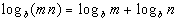m > 0 and n > 0

 Basically, what we are saying here is that another way to write the log of a product is to take the log of the first base and add it to the log of the second base.   Hmmmm, why don’t I just take the product of their logs?????? Wait a minute, I remember my teacher saying above that logs are another way to write exponents - WHENEVER  I WAS MULTIPLYING LIKE BASES, I ADDED MY EXPONENTS - SO I’M GOING TO HAVE TO ADD MY LOGS - EUREKA!!!!  Note that even though m and n are not the bases of the log itself, they can each be written as base b to an exponent, because of the definition of logarithms.

 Here is a quick illustration of how this property works: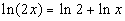Property 2 Quotient Rulem > 0 and n > 0

 Basically, what we are saying here is that another way to write the log of a quotient is to take the log of the numerator and subtract the log of the denominator.    So here, we have to remember that when we were dividing like bases, we subtracted our exponents - so we do the same type of thing with our logs.

 Here is a quick illustration of how this property works: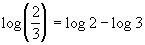Property 3 Power Rule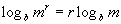m > 0

 Basically, what we are saying here is that whenever you have a 2nd exponent inside the log - remember the log itself is an exponent - then you can pull it out front and multiply it times the log.   Wow, that looks a little different, but again it comes from the fact that logs are another way to write exponents.  Remember that when we had a base raised to 2 powers that we would multiply those 2 exponents together.  That is what we are doing here.  Again, even though m is not the base of the log, it can be written as b to an exponent (based on the log definition) and the log itself is an exponent so we have a double exponent - so we multiply our exponents together.

 Here is a quick illustration of how this property works: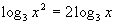Property 4 Change of Base Formula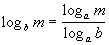m > 0 and b > 0

 Basically, what we are saying here is, whenever you need to change the base of your log you can rewrite it as the log base a (whatever the new base is) of the original inside over the log base a (whatever the new base is) of the original base.   Your calculator is limited to only finding base 10 and base e logarithms.  That would leave us in a bind if we needed to find the value of a log with any other base.  So we can use this change-of-base formula to change it to base 10 or e so we could find a value.  Neat, huh?

 Here is a quick illustration of how this property works: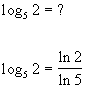Example 1: Expand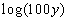as much as possible.  Evaluate without a calculator where possible.

 Note how there is no base written.  Does that mean there is no base?  Not in the least.  What would the base be in this problem?  If you said 10 you are correct. This is known as the common log.  If you need a review on the common log (log base 10), feel free to go to Tutorial 43: Logarithmic Functions.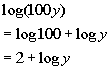*Use the product rule *Use the definition of logs to simplify *2 is the exponent needed on 10 to get 100Example 2: Expand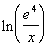as much as possible.  Evaluate without a calculator where possible.

 Note how there is a ln and no base written.  What would the base be in this problem?  If you said e you are correct. This is known as the natural log.  If you need a review on the natural log (log base e), feel free to go to Tutorial 43: Logarithmic Functions.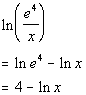*Use the  quotient rule *Use the definition of logs to simplify *4 is the exponent needed on e to get e^4Example 3: Expand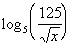as much as possible.  Evaluate without a calculator where possible.

 What is the base in this problem? This time the base is 5.  Make sure that you keep that same base throughout the problem.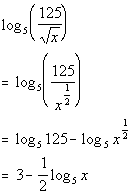*Use the quotient rule *Use the power rule   *Use the definition of logs to simplify *3 is the exponent needed on 5 to get 125Example 4: Expand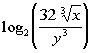as much as possible.  Evaluate without a calculator where possible.

 What is the base in this problem? This time the base is 2.  Make sure that you keep that same base throughout the problem.*Use the product rule and the quotient rule *Use the definition of logs to simplify *5 is the exponent needed on 2 to get 32 *Use the power ruleExample 5: Condense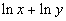into one logarithmic expression.  Evaluate without a calculator where possible.

 This time we are going in reverse of what we did in examples 1 - 4.  However, you can use the same properties we used on them.  You can use those properties in either direction. What is the base in this problem? This time the base is e.  Make sure that you keep that same base throughout the problem.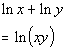*Use the product ruleExample 6: Condense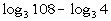into one logarithmic expression.  Evaluate without a calculator where possible.

 Again we are going in reverse of what we did in examples 1 - 4.  What is the base in this problem? This time the base is 3.  Make sure that you keep that same base throughout the problem.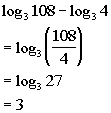*Use the quotient rule   *Use the definition of logs to simplify *3 is the exponent needed on 3 to get 27Example 7: Condense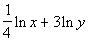into one logarithmic expression.  Evaluate without a calculator where possible.

 Again we are going in reverse of what we did in examples 1 - 4.  What is the base in this problem? This time the base is e.  Make sure that you keep that same base throughout the problem.*Use the power rule   *Use the product ruleExample 8: Rewrite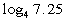using natural logarithms and evaluate using a calculator.  Round to 4 decimal places.

 Note that the calculator can only evaluate logs that are base 10 or base e.  Since this problem is in base 4,  we need to change the base to base e (or base 10).  Since the instructions say base e, let's do that. This should land between 1 and 2, because 7.25 lands between 4^1 = 4 and 4^2 = 16.  Again logs are another way to write exponents and that is what we are looking for here.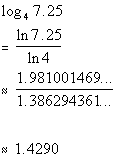*Use the calculator to find ln 7.25 and ln 4   *DividePractice Problems

 These are practice problems to help bring you to the next level.  It will allow you to check and see if you have an understanding of these types of problems. Math works just like anything else, if you want to get good at it, then you need to practice it.  Even the best athletes and musicians had help along the way and lots of practice, practice, practice, to get good at their sport or instrument.  In fact there is no such thing as too much practice. To get the most out of these, you should work the problem out on your own and then check your answer by clicking on the link for the answer/discussion for that  problem.  At the link you will find the answer as well as any steps that went into finding that answer.Practice Problems 1a - 1c:  Expand each logarithmic expression as much as possible.  Evaluate without a calculator where possible.

 1a.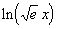(answer/discussion to 1a) 1b.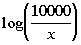(answer/discussion to 1b)

 1c.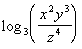(answer/discussion to 1c)Practice Problems 2a - 2b: Condense each logarithmic expression into one logarithmic expression.  Evaluate without a calculator where possible.

 2a.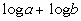(answer/discussion to 2a) 2b.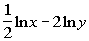(answer/discussion to 2b)Practice Problem 3a:  Rewrite the logarithmic expression using natural logarithms and evaluate using a calculator.  Round to 4 decimal places.

 3a.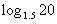(answer/discussion to 3a)Need Extra Help on these Topics?

The following are webpages that can assist you in the topics that were covered on this page.

 http://www.purplemath.com/modules/logrules.htm This webpage helps you with logarithmic properties. http://www.sosmath.com/algebra/logs/log4/log43/log43.html This webpage helps explain the change of base formula.

Go to Get Help Outside the Classroom found in Tutorial 1: How to Succeed in a Math Class for some more suggestions.

Last revised on March 23, 2011 by Kim Seward.